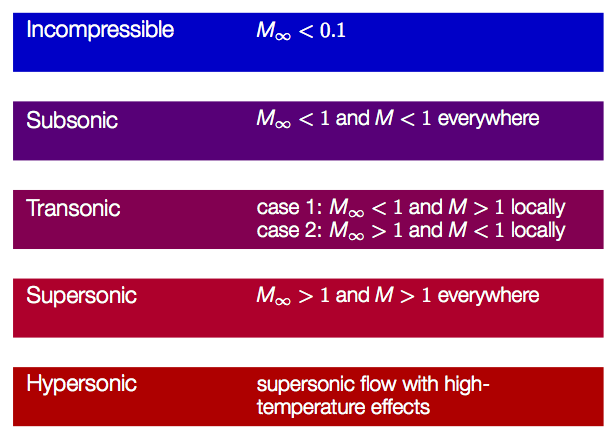## Chapter 1

Compressible Flow

## Compressible Flow

### Overview

This first chapter gives an overview of the field of compressible flow. In the first sections important milestones in the compressible flow history are presented. Concepts and parameters relevant for compressible flows are introduced and discussed. A short review of thermodynamics is given at the end of the chapter.

### Online Flow Calculator

Sections of the online flow calculator for compressible flow problems CFLOW related to this chapter:

• Section 1: Gas Relations

### Sections

#### 1.2 Definition of Compressible Flow

This chapter discusses the difference between compressible and incompressible flow. The concept compressibility is defined and discussed.

#### 1.3 Flow Regimes

The free stream and local Mach numbers are defined as $$M_\infty=V_\infty/a_\infty$$ and $$M=V/a$$, respectively. The Mach number is used to define different regimes of compressible flows (subsonic, transonics, supersonic, hypersonic). The concept inviscid flows is introduced.Figure 1: Compressible flows can be divided into flow regimes based on the local flow Mach number and the free stream Mach number

#### 1.4 A Brief Review of Thermodynamics

This chapter gives, as the title hints, a short review of thermodynamics. Concepts important for compressible flows are introduced:

• perfect gas
• equation of state (ideal gas law) $$p=\rho RT$$
• internal energy $$e$$
• enthalpy $$h=e+p/\rho$$
• thermally perfect gas
• callorically perfect gas
• specific heat
$$C_p=\left(\frac{\partial h}{\partial T}\right)_p$$
$$C_v=\left(\frac{\partial e}{\partial T}\right)_v$$
$$C_p-C_v=R$$
$$\gamma=\frac{C_p}{C_v}$$
$$C_p=\frac{\gamma R}{\gamma-1}$$
$$C_v=\frac{R}{\gamma-1}$$

The first law of thermodynamics is introduced and described.

$$\delta q + \delta w =de$$

Three type of processes are introduced:

• reverisible
• isentropic

The concept of entropy is introduced and discussed in the framework of the second law of thermodynamics. Calculation of entrpoy finally leads us to the isentropic relations, which will prove to be very useful throughout the rest of the book.

$$\frac{p_2}{p_1}=\left(\frac{\rho_2}{\rho_1}\right)^{\gamma}=\left(\frac{T_2}{T_1}\right)^{\gamma/(\gamma-1)}$$

#### Aerodynamic Forces on a Body

This section describes how forces on a body immersed in a fluid flow can be calculated. As an example, a formula for the evaluation of aerodynamic forces on a wing is derived and in conjunction with that the force components lift and drag are introduced.

### Study Guide

The questions below are intended as a "study guide" and may be helpful when reading the text book.

1. How are the compressibility factors $$\tau_T$$ and $$\tau_S$$ defined?
2. For a given flow, how can we determine if compressibility effects are important?
3. Use the Bernoulli equation (even though it's not valid for compressible flows) to obtain an estimate of the Mach number for which compressible effects becomes significant and must be considered.
4. What are the criteria for the classifications subsonic/transsonic/supersonic/hypersonic flow?
5. What is a perfect gas and what relation between pressure, density, temperature may be used for such a gas?
6. What do we mean by thermally perfect gas and calorically perfect gas respectively?
7. When can air be regarded as a calorically perfect gas?
8. Define $$C_p$$, $$C_v$$, and $$\gamma$$
9. In the first law of thermodynamics, how is a {\bf{system}} defined?
10. What is a reversible process?
11. What is an adiabatic process?
12. What are the criteria for an isentropic process, i.e. what conditions must be satisfied for a steady-state compressible flow to be isentropic?
13. How do we define entropy for a gas?
14. What does the second law of thermodynamics say about entropy?
15. Derive the isentropic relations for calorically perfect gases starting from the entropy equation.
16. In Formulas, Tables & Graphs, check all thermodynamics formulas for thermally perfect gas and calorically perfect gas on the first three pages. You should be familiar with these formulas and know when/how to apply them.